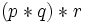# Group extension operator

This is a binary group property operator, viz an operator that takes as input two group properties, and outputs one group property

BEWARE! This term is nonstandard and is being used locally within the wiki. [SHOW MORE]

## Definition

### Symbol-free definition

The group extension operator is a binary operation on the group property space. It takes in two group properties$p$ and$q$ and returns the property of being a group extension of a group with property$p$ by a group with property$q$, or in other words, a group that has a normal subgroup with group property$p$, whose quotient satisfies group property$q$.

### Definition with symbols

The group extension operator s a binary operation on the group property space. Given two group properties$p$ and$q$ the group extension property$p$ by$q$ is defined as the property that is satisfied by a group$G$ if and only if$G$ has a normal subgroup$N$ such that:

•$N$ satisfies property$p$ as an abstract group.
• The quotient group$G/N$ satisfies property$q$ as an abstract group.

## Property theory

### Associativity

Although the group extension operator is not associative, it satisfies forward associativity, namely:$p * (q * r)$$(p * q) * r$

### Commutativity

The group extension operator is far from commutative.

### Transitivity

As for any other binary property operator, we can define the notion of transitivity for the group extension operator, and also the notion of t.i. group property (here, the identity is the property of being the trivial group). However, because the group extension operator is only forward associative, only some of the results of the transiter master theorem remain valid. In particular, the following are true:

• Any transitive property implies its left (respectively right) transiter.
• Any identity-true property is implied by its left (respectively right) transiter.
• Thus, any t.i. property equals its left transiter as well as its right transiter.
• Both the left and the right transiter of any property are identity-true.
• The right transiter of any property is transitive. This follows from the forward associativity.

Note that the last of the statements is the only one where associativity is used.

Put together, these observations tell us that the right transiter operator with respect to the group extension operator is an idempotent operator with its fixed point cum image space being precisely the t.i. group properties with respect to the extension operator, that is, those properties which are satisfied by the trivial group and closed under extensions.

The left transiter, on the other hand, is far more murky.

### Subordination

Because of forward associativity of the group extension operator, the smallest t.i. property that implies a given property can be obtained as the Kleene-star closure with respect to left-associated powers.